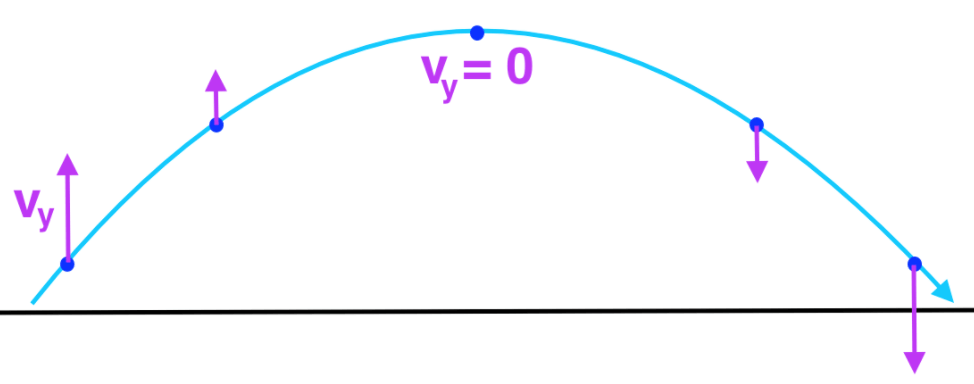# Vertical Velocity Calculator

Put the required values in the boxes below to find initial vertical velocity or vertical velocity at time using vertical motion calculator.

m/s
m/s2
s## Formula

Vertical Velocity at Time:
Vy = Vy0 - gt

Initial Vertical Velocity:
Vy0 = Vy + gt

Acceleration of Gravity:
g = Vy0 - Vy /t

Time:
t = Vy0 - Vy / g

Where,
Vy = Vertical Velocity at Time
Vy0 = Initial Vertical Velocity
g = Acceleration of Gravity
t = Time.

The vertical component of velocity calculator uses above equations to calculate the vertical velocity at time and initial vertical velocity.

Initial vertical velocity calculator is an online tool that proficiently finds the vertical velocity of the object in projectile motion. The projectile velocity calculator calculates the:

• Vertical velocity at time
• Initial vertical velocity
• Acceleration of gravity, and
• Time

In next sections, we will elaborate vertical velocity definition, vertical velocity equations, and how to find vertical velocity without using vertical acceleration calculator.

## What is projectile motion?

Projectile motion is the motion of a projectile object.

A projectile is an object upon which the only force acting is gravity. There are a variety of examples of projectiles: an object dropped from rest is a projectile provided that the influence of air resistance is negligible.

### What is vertical velocity of projectile motion?

The vertical component of a projectile's velocity is subject to the force of gravity. It accelerates downward resulting in greater distances that are covered in each successive time interval.## How to calculate vertical velocity at time?

Done exploring horizontal and vertical velocity calculator? It’s time to fold up your sleeves, because we are going to explain the manual method to calculate vertical velocity with an example.

Example:

Calculate the vertical velocity at time for a projectile object with an initial vertical velocity of 40 m/s, having acceleration of gravity of 10 m/s2 in 2 s.

Solution:

Step 1: Identify and write down the values.

Vy0 = 40 m/s

g = 10 m/s2

t = 2 s

Step 2: Use the formula of vertical velocity at time and place the values.

Vy = Vy0 – gt
Vy = 40 – 10 × 2
Vy = 40 – 20
Vy = 20 m/s

Vertical Velocity at Time (Vy) = 20m/s

You can simply place the values of above example in projectile acceleration calculator to directly get the answer.

1. Vertical Velocity |. Six Flags.
2. Vertical Velocity - an overview | ScienceDirect Topics.